Select Page

# CBSE Maths 12 Science Three Dimensional Geometry MCQ Answers in English

CBSE Maths 12 Science Three Dimensional Geometry MCQ Answers in English to enable students to get Answers in a narrative video format for the specific question.

Expert Teacher provides CBSE Maths 12 Science Three Dimensional Geometry MCQ Answers through Video Answers in English language. This video solution will be useful for students to understand how to write an answer in exam in order to score more marks. This teacher uses a narrative style for a question from Three Dimensional Geometry not only to explain the proper method of answering question, but deriving right answer too.

Please find the question below and view the Answer in a narrative video format.

Question:

## Similar Questions from CBSE, 12th Science, Maths, Three Dimensional Geometry

Question 1 : If a line makes angle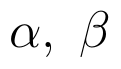and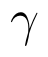with the coordinate axis, then find the value of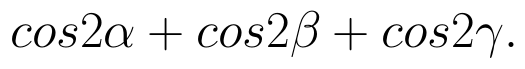(View Answer Video)

Question 2 : Write the distance between the parallel planes 2x - y + 3z = 4 and 2x - y + 3z = 18. (View Answer Video)

Question 3 : Find the distance between the point (-1, -5, -10) and the point of intersection of line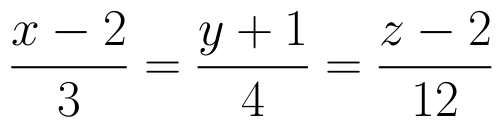and plane

Question 4 : Find the distance of the point (1, -2, 3) from the plane x-y+z=5 measured parallel to the line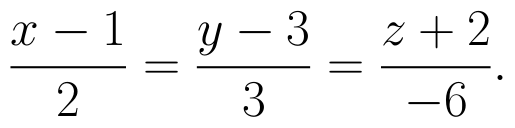(View Answer Video)

Question 5 : Find the co-ordinates of the point where the line through the points A (3, 4, 1) and B (5, 1, 6) crosses the XY-plane. (View Answer Video)

### Linear Programming

Question 1 : The objective function is maximum or minimum, which lies on the boundary of the feasible region. (View Answer Video)

### Matrices

Question 1 : Let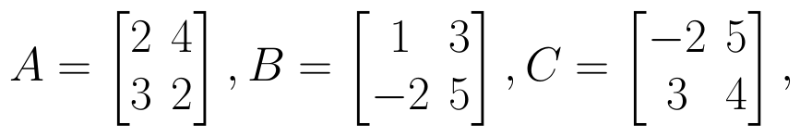Find A - B. (View Answer Video)

Question 2 : Compute: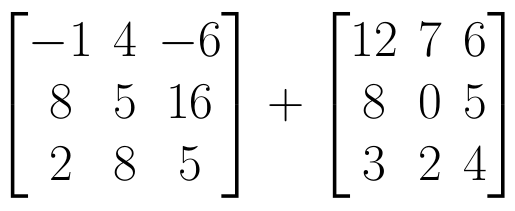. (View Answer Video)

Question 3 : Find the value of Y, if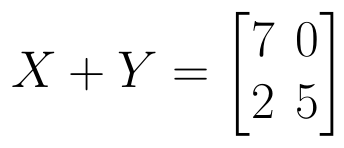and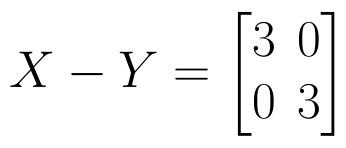. (View Answer Video)

Question 4 : Find the value of x,  from the equation: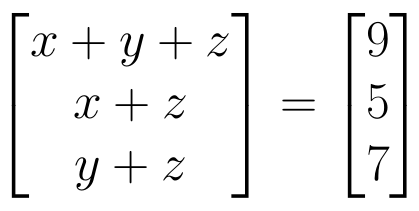. (View Answer Video)

Question 5 : If for any square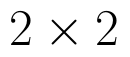matrix A,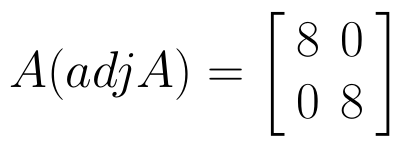then write the value of |A|. (View Answer Video)

### Integrals

Question 1 : Evaluate: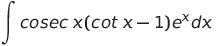(View Answer Video)

Question 2 : Find the integral of the function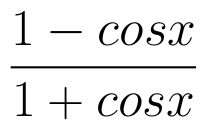. (View Answer Video)

Question 3 : Prove that :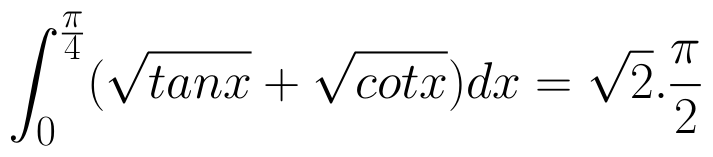(View Answer Video)

Question 4 : Evaluate :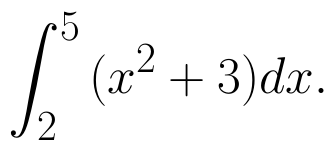(View Answer Video)

Question 5 : Find: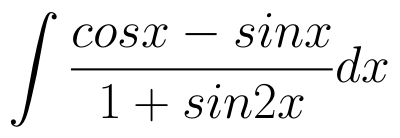. (View Answer Video)